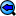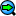Hex scattering database
Input:
Choose what to compute:
Choose units:
Energy: , Angles: , Output:
Set initial atomic state(s):
$n_i$ = $l_i$ = $m_i$ =
Set final atomic state(s):
$n_f$ = $l_f$ = $m_f$ =
Set uniform energy range:
$E_{\mathrm{min}}$ = $E_{\mathrm{max}}$ = $\Delta E$ =
or
retrieve all available energies.
Graph axis scales:
Horizontal:     Vertical:

Output:
This section contains a graphical preview of the selected data. It uses Gnuplot's HTML5 Canvas output, which allows a trivial interaction. You can zoom in using right or middle button (browser-dependent) or toggle the plots on and off. Old browsers will not display the graphs correctly.
Sorry, your browser seems not to support the HTML 5 canvas element.➀ ➁ x y